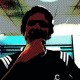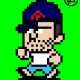# Plotting pitches – ggplot2 tips and tricks

In Chapter 6 of our book we presented a step-by-step guide for producing plots with the powerful `ggplot2` package.

While you can get a close-to-finished `ggplot2` plot without much hassle, sometimes the finishing touch may require more time than one would expect (and even get you some frustrations when you don’t understand why an error occurs or something isn’t shown as expected).

Here I will start showing a few tips and tricks to finish up your `ggplot2` figures.

Let’s first get us some data to plot. I will make examples using pitches thrown by Clayton Kershaw on Opening Day 2013, when he completed a 94-pitches shutout.
Just like Jim did in the previous post, I’ll make use of the `pitchRx` package to get the desired data. Notice that data are grabbed as strings, thus conversions to numeric are required for some columns before plotting.

```library(pitchRx)

# get data from Apr. 1, 2013
dat = scrapeFX(start="2013-04-01", end="2013-04-01")

pitches = plyr::join(dat\$pitch, dat\$atbat,
by = c("num", "url"), type = "inner")

# Clayton Kershaw's pitches
kershaw = subset(pitches, pitcher_name == "Clayton Kershaw")

# convert characters to numbers
kershaw\$px = as.numeric(kershaw\$px)
kershaw\$pz = as.numeric(kershaw\$pz)
kershaw\$start_speed = as.numeric(kershaw\$start_speed)
```

As I said, you can get your first plot very easily.

```library(ggplot2)

# basic example
ggplot(data=kershaw, aes(x=px, y=pz, shape=type, col=pitch_type)) +
geom_point()
```

With the code above, we indicate that `kershaw` is the data frame to be used, that the variables `px` and `pz` are to be mapped to the x-axis and y-axis respectively, then `type` will affect shape and `pitch_type` color. Here’s the result.

By just adding a couple of lines of code, we can have side-by-side plots with data split by opponent handedness, and equal coordinates since `px` and `pz` are expressed in the same unit.

```# add faceting, equal coordinates
ggplot(data=kershaw, aes(x=px, y=pz, shape=type, col=pitch_type)) +
geom_point() +
facet_grid(. ~ stand) +
coord_equal()
```

Now, like we did in the example in the book, we’d like to add a strike zone box. As a first step we prepare a data frame containing the coordinates needed to draw the path.

```topKzone = 3.5
botKzone = 1.6
inKzone = -.95
outKzone = 0.95
kZone = data.frame(
x = c(inKzone, inKzone, outKzone, outKzone, inKzone)
, y = c(botKzone, topKzone, topKzone, botKzone, botKzone)
)
```

Unfortunately, when we add the `geom_path` call, we get an error and no strike zone is plotted.

```ggplot(data=kershaw, aes(x=px, y=pz, shape=type, col=pitch_type)) +
geom_point() +
facet_grid(. ~ stand) +
coord_equal() +
geom_path(aes(x, y), data = kZone)
```

`Error in eval(expr, envir, enclos) : object 'type' not found`

The reason for this error is that on the main `ggplot` call (first line) we passed aesthetics for shape and color, thus such aesthetics are expected in every subsequent call, included the `geom_path` we just added.
To avoid this problem, we do not pass any argument to the main `ggplot` call, moving everything in the `geom_point` call in the second line.
The following code returns the expected result.

```ggplot() +
geom_point(data=kershaw, aes(x=px, y=pz, shape=type, col=pitch_type)) +
facet_grid(. ~ stand) +
coord_equal() +
geom_path(aes(x, y), data = kZone)
```

In the next steps I’ll show how to make small changes to finalize the plot.
First we store the plot as was created in the last code chunk in an R object named `p0`.

```p0 = ggplot() +
geom_point(data=kershaw, aes(x=px, y=pz, shape=type, col=pitch_type)) +
facet_grid(. ~ stand) +
coord_equal() +
geom_path(aes(x, y), data = kZone)
```

Then we begin by modifying the axis labels, which is pretty straightforward.

```p1 = p0 +
xlab("horizontal location\n(ft. from center of the plate)") +
ylab("vertical location\n(ft. from ground)")
p1
```

As a second step we modify the color legend: the legend title is changed to “pitch type” (`name` argument), the pitch type abbreviations are substituted with full names (`labels` argument). Also different colors are chosen (`values` argument).
Note that, since we acted on the color legend, we called the `scale_color_manual` function.

```p2 = p1 +
scale_color_manual(name="pitch type"
, values=c("CU"="blue", "FF"="red", "SL"="black")
, labels=c("CU"="Curveball", "FF"="4-seam Fastball", "SL"="Slider"))
p2
```

Similarly, the shape part of the legend can be modified as follows.

```p3 = p2 +
scale_shape_manual(name="outcome"
, values=c("B"=0, "S"=1, "X"=4)
, labels=c("B"="ball", "S"="strike", "X"="ball in play"))
p3
```

The plot below is the results of the changes apported to both the color and shape legends.

Let’s say we want to add an entry to the legend to tell people looking at the plot that the box indicates the rulebook strike zone. In order to have something shown in a `ggplot2` legend, it has to be passed as an aesthetic: that’s what happens in the plot shown above with the pitch type (passed as the color aesthetic) and outcome (passed as the shape aesthetic).

To obtain what we are looking for, we slightly change the `p0` object.

```p0 = ggplot() +
geom_point(data=kershaw, aes(x=px, y=pz, shape=type, col=pitch_type)) +
facet_grid(. ~ stand) +
coord_equal() +
geom_path(aes(x, y, linetype="kzone"), data = kZone)
```

Note that, in this case, we passed a constant string to the `linetype` aesthetic.
Let’s now re-run the code to obtain `p3`.

```p1 = p0 +
xlab("horizontal location\n(ft. from center of the plate)") +
ylab("vertical location\n(ft. from ground)")

p2 = p1 +
scale_color_manual(name="pitch type"
, values=c("CU"="blue", "FF"="red", "SL"="black")
, labels=c("CU"="Curveball", "FF"="4-seam Fastball", "SL"="Slider"))

p3 = p2 +
scale_shape_manual(name="outcome"
, values=c("B"=0, "S"=1, "X"=4)
, labels=c("B"="ball", "S"="strike", "X"="ball in play"))
```

And then we finish up the line type entry in the legend using commands analogous to the ones used for shape and color.

```p4 = p3 + scale_linetype_manual(name=""
, values=c("kzone"=2)
, labels=c("kzone"="rulebook K-zone"))
p4
```

As a final touch to the plot, we want to modify the labels on the strips, changing “L” and “R” with “vs. LHB” and “vs. RHB” respectively.
The most straightforward way to achieve that is to simply modify your data frame, so that you have the proper labels in the `stand` column (or in a new one).
However, if you have reasons for not wanting to mess with your data frame, here’s a way (albeit a bit awkward) to obtain the desired result.

Three steps need to be performed.
First, a list has to be built, which maps values in the data frame to desired labels.

```opp_hand <- list("L" = "vs. LHB", "R" = "vs. RHB")
```

Then the following “labeller” function is created

```opp_hand_labeller <- function(variable,value){
return(opp_hand[value])
}
```

Finally, the function above is passed to the `labeller` argument in the `facet_grid` call.

```p0 <- ggplot() +
geom_point(data=kershaw, aes(x=px, y=pz, shape=type, col=pitch_type)) +
facet_grid(. ~ stand, labeller=opp_hand_labeller) +
coord_equal() +
geom_path(aes(x, y, linetype="kzone"), data = kZone)
```

Then it’s just a matter of re-running the code previously shown until you have the new version of the `p4` object.

```p1 = p0 +
xlab("horizontal location\n(ft. from center of the plate)") +
ylab("vertical location\n(ft. from ground)")

p2 = p1 +
scale_color_manual(name="pitch type"
, values=c("CU"="blue", "FF"="red", "SL"="black")
, labels=c("CU"="Curveball", "FF"="4-seam Fastball", "SL"="Slider"))

p3 = p2 +
scale_shape_manual(name="outcome"
, values=c("B"=0, "S"=1, "X"=4)
, labels=c("B"="ball", "S"="strike", "X"="ball in play"))

p4 = p3 + scale_linetype_manual(name=""
, values=c("kzone"=2)
, labels=c("kzone"="rulebook K-zone"))
p4
```

### 8 responses

1.Just found out about your book and ordered it through the publisher. I’m huge into sabermetrics and am tech savvy, yet R has given me so much aggravation. I’m hopeful the text allows me to really understand the basics of R and the installation of packages and datasets that I can use on a regular basis. I use analytics regularly in my baseball writing, so this is great news. I’m excited.

Also,I believe Albert was a Temple grad … fellow Owl here, too.

2.We hope you find the book helpful. I understand that R has a steep learning curve ( I work with a lot of students), but I think once you get a certain comfort level, it goes pretty well. By the way, although I grew up in Philly, Jay Bennett (my Curve Ball coauthor) is the Temple grad.

3.That’s right, it was Jay. Curve Ball is one those books in my library I refer to almost weekly. Where in Philly did you grow up, Jay? I was down in Juniata and went to North Catholic …

1.I grew up in Lafayette Hill (Whitemarsh). I still make regular visits to Philly to see my mom. I went to graduate school in Indiana and didn’t make it make to PA. Thanks for the comments about Curve Ball.

4.Hi Jim and Max,

I’m loving these posts! I think this post in particular is a great way to learn some of the quirks of ggplot2. I just wanted to note that pitchRx::strikeFX() has support for automatically drawing strike-zones as described by Mike Fast:

http://www.baseballprospectus.com/article.php?articleid=14572

It’s also worth noting you can always use “ggplot arithmetic” with strikeFX if you want to add layers or customize the output. For example,

library(pitchRx)
data(pitches)
strikeFX(pitches)+facet_grid(.~stand)+theme_bw()

That said, I’m most excited about the model option of strikeFX – which is still under development. The basic idea is to ease the process behind visualizing a Generalized Additive Model (fit using the mgcv package). The newest version of pitchRx has some examples on the strikeFX help page. If you have any questions or feedback, please let me know!

5.This might be a dumb question, but are these charts from the catcher’s perspective? In general, are the px and pz values from the catcher’s or pitcher’s perspective? As in negative px values would be inside to RHB?

Thanks!

Isaac

6.It’s not a dumb question Isaac: one should make charts self-explanatory and I didn’t!
Yes the charts are from the catcher’s perspective, and usually (but not necessarily) that’s how they are shown. And per PITCHf/x, negative px is inside to RHB.

7. […] Now it’s time to use R’s graphic engine, with the help of ggplot2 package (see some ggplot2 tricks here). […]